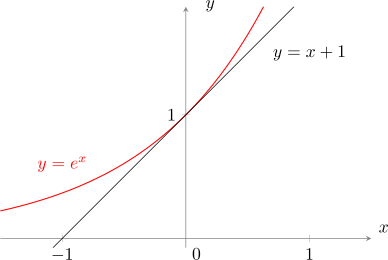En esta pagina encontraras material relacionado a activades docentes que son de utilidad con alguna de las asignaturas o cursos que dicto.

A height-diameter model has the following structure $\begin{equation} h_i=f({\theta},d_i) + \epsilon_i, \label{eq:modh.0} \end{equation}$
where: $$h_i$$ is height at the $$i$$-th tree; $$d_i$$ is diameter at breast height at the $$i$$-th tree; $$f()$$ is a mathematical function; $${\theta}$$ is a vector of coefficients (i.e., parameters) of model $$f()$$; $$\epsilon_{i}$$ is the random term for the $$i$$-th tree following a Gaussian probability density function having an expected value of zero and variance $$\sigma^2_{\epsilon}$$. Noice that function $$f()$$ could either be linear o non-linear.